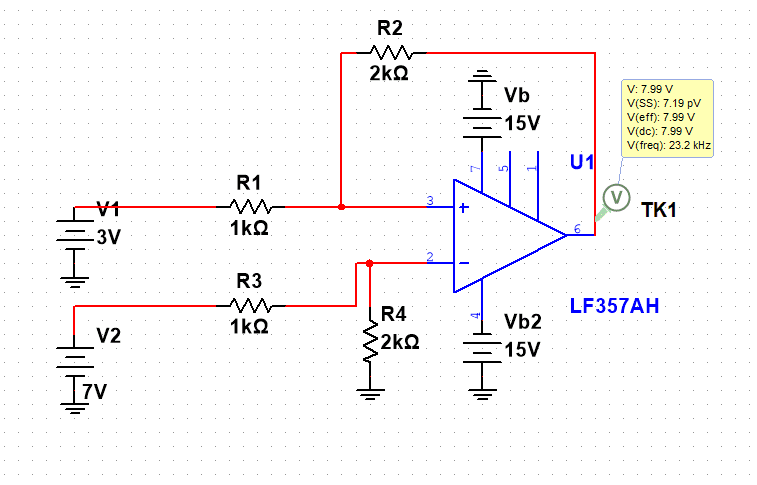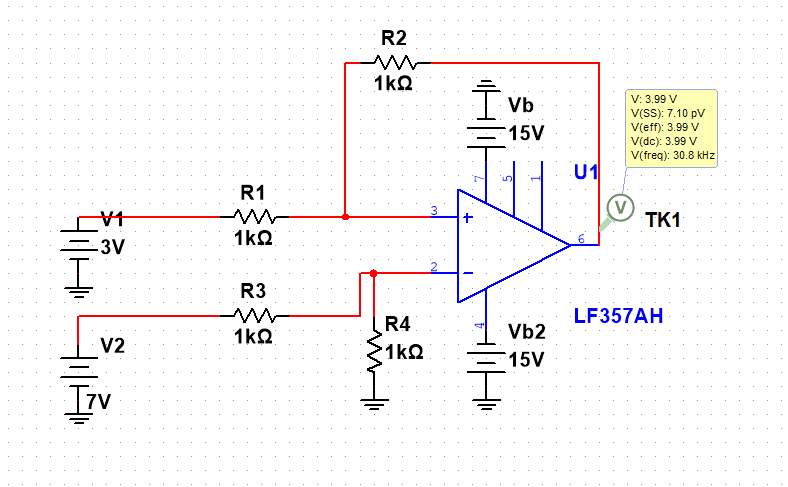# Opamp subtractor circuit

• Engineering
• altruan23
In summary, the conversation discusses the use of superposition and setting the ratio of resistors in a circuit to achieve a subtractor. The book "Foundations of Analog and Digital Electronics" by Agarwal and Lang explains that this circuit is a subtractor, but the speaker questions whether it is actually a differential amplifier. They then go on to simulate both circuits and conclude that all resistors must be the same for a true subtractor. The book is referenced for more information on the topic. The conversation ends with a discussion on the difference between a differential amplifier and a subtractor.f

#### altruan23

Homework Statement
So i was learning about the subtractor op amp circuit and saw that everyone is saying that a subtractor is the same as a differential op amp.
Relevant Equations
superposition, voltage divider , op amp
So after using superposition and setting the ratio R2/R1 = R4/R3 the same or R2=R4 and R1=R3,i come to the eq. for output voltage Vout= R2/R1 * (V2-V1) or R4/R3(V2-V1). And in the book foundations of analog and digital electronics by agarwal and lang, they are saying that this circuit is a subtractor.
but isn't that just an differential amplifier, as it amplifies with the ratio R2/R1 the difference (V2-V1)? Schouldnt a subtractor be Vout= V2-V1 so that means i need to have R1=R2=R3=R4. why is everyone saying that a differential amp. is the same as subtracter?? i simulated both circuits and as i tought, all resistors must be the same to really have a subtractor.
The first picture is R2/R1 = R4/R3 as in the book.So as you can see, 7V-3V schould be 4 according to a real subtractor, but because the ratio R2/R1 or R4/R3 is 2, Uout is 2*4=8.
And if i set all the resistors the same i truly get a real subtractor.So why is everyone saying that a differential amp is the same as subctractor?? i mean a subtractor is a differential amp with gain 1, but a differential amp with some other gain isn't a subtractor.

the book is here: https://neurophysics.ucsd.edu/courses/physics_120/Agarwal and Lang (2005) Foundations of Analog and Digital.pdf
online book page 883

So why is everyone saying that a differential amp is the same as subctractor?? i mean a subtractor is a differential amp with gain 1, but a differential amp with some other gain isn't a subtractor.
If the term (V2 - V1) is present then it is subtracting two voltages, by computing a difference. The gain is a bonus since the output can be scaled without adding more resistors or another op-amp. In analog electronics everything is scaled to use the available common mode range.

•altruan23